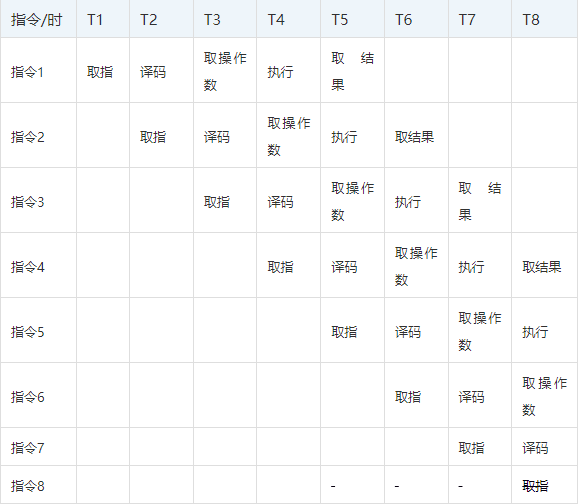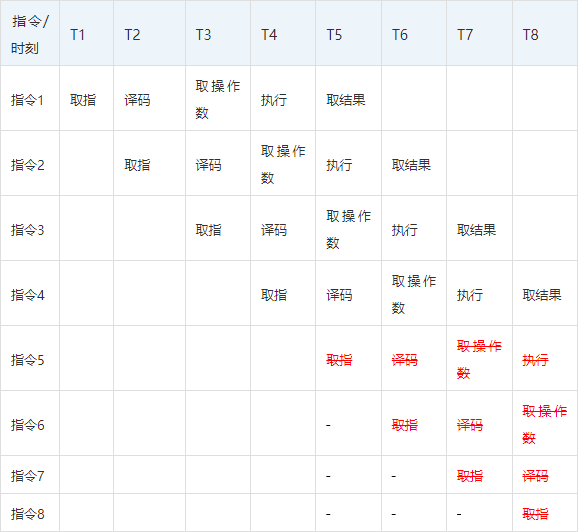# 为什么新一代的Rust、Go等编程语言都如此讨厌if-else、Switch结构

Swich的坑，环境一变效率就差远了

package main import( "fmt" "math/rand" //"sync" "time" ) funcmain{ now := time. Now. UnixNano count:= []int{ 0, 0, 0, 0, 0, 0} fori := 0; i < 100000; i++ { random := rand. Intn( 100) switchrandom { case1: count[ 1]++ case2: count[ 2]++ case3: count[ 3]++ case4: count[ 4]++ case5: count[ 5]++ default: count[ 0]++ } } fmt. Println(time. Now. UnixNano- now) fmt. Println( count) }

[root@ecs-a4d3 hello_world]# time ./test1 288084 [99507 86 108 106 96 97] real 0m0.004s user 0m0.003s sys 0m0.001s

package main import( "fmt" "math/rand" //"sync "time" ) funcmain{ now := time. Now. UnixNano count:= []int{ 0, 0, 0, 0, 0, 0} fori := 0; i < 100000; i++ { random := rand. Intn( 1000) switchrandom { case1: count[ 1]++ case2 count[ 2]++ case3: count[ 3]++ case4: count[ 4]++ case5: count[ 5]++ default: count[ 0]++ } } fmt. Println(time. Now. UnixNano- now) fmt. Println( count) }

[root@ecs-a4d3 hello_world]# time ./test1 4365712 [20184 20357 19922 19897 19640 0] real 0m0.006s user 0m0.004s sys 0m0.002s

CPU流水线与指令预测default: count[ 0]++ 49a0f9: 488b 442460mov 0x60(%rsp),%rax 49a0fe: 48ff 00inc q (%rax) 49a101: eb 84jmp 49a087 <main.main+ 0xa7> case 3: 49a103: 4883f8 03cmp \$0x3,%rax 49a107: 750e jne 49a117 <main.main+ 0x137> count[ 3]++ 49a109: 488b 442460mov 0x60(%rsp),%rax 49a10e: 48ff 4018incq 0x18(%rax) 49a112: e9 70ff ff ff jmpq 49a087 <main.main+ 0xa7> case 4: 49a117: 4883f8 04cmp \$0x4,%rax 49a11b: 750e jne 49a12b <main.main+ 0x14b>

Rust的if else 也是一样的坑

use rand::Rng; fn main { let mut rng = rand::thread_rng; let mut arr = [0, 0, 0, 0, 0,0]; //println!("Integer: {}", arr[random]); for i in 0..1000000 { let mut random =rng.gen_range(0,5); if random==0{ arr=arr+1; } else if random==1{ arr=arr+1; } else if random==2{ arr=arr+1; } else if random==3{ arr=arr+1; } else if random==4{ arr=arr+1; } else{ arr=arr+1; } } println!("{},{},{},{},{},{}", arr, arr, arr, arr, arr, arr); }

letmut random =rng.gen_range( 0, 100);

packagemain import( "fmt" "math/rand" "time" ) funcmain{ now := time.Now.UnixNano //count := []int{0, 0, 0, 0, 0, 0} count := map[ int] int{ 0: 0, 1: 0, 2: 0, 3: 0, 4: 0, 5: 0} fori := 0; i < 100000; i++ { random := rand.Intn( 5) count[random]++ } fmt.Println(time.Now.UnixNano - now) fmt.Println(count) }

[root@ecs-a4d3 hello_world]# time ./test2 5864780 map[ 0: 201841: 203572: 199223: 198974: 196405: 0] real0m0 .008s user 0m0 .007s sys 0m0 .001s

END